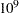The OPTMILP Procedure
 ODS Tables

PROC OPTMILP creates two Output Delivery System (ODS) tables by default unless you specify a value other than 1 for the PRINTLEVEL= option. The first table, "ProblemSummary," is a summary of the input MILP problem. The second table, "SolutionSummary," is a brief summary of the attempt to solve the problem. You can refer to these tables when using ODS. An example output of PROC OPTMILP is shown in Figure 18.5 (Problem Summary) and Figure 18.6 (Solution Summary). For more information about ODS, see SAS Output Delivery System: User’s Guide.

If you specify a value of 2 for the PRINTLEVEL= option, then a third table, "ProblemStatistics," is produced. This table contains information about the problem data. See the section Problem Statistics for more information.

Table 18.11 ODS Tables Produced by PROC OPTMILP

ODS Table Name

Description

PRINTLEVEL=

ProblemSummary

Summary of the input MILP problem

1 (default)

SolutionSummary

Summary of the solution status

1 (default)

ProblemStatistics

Description of input problem data

2

Figure 18.5 Example PROC OPTMILP Output: Problem Summary

The OPTMILP Procedure

Problem Summary
Problem Name EX_MIP
Objective Sense Minimization
Objective Function COST
RHS RHS

Number of Variables 3
Bounded Above 0
Bounded Below 0
Bounded Above and Below 3
Free 0
Fixed 0
Binary 3
Integer 0

Number of Constraints 3
LE (<=) 2
EQ (=) 0
GE (>=) 1
Range 0

Constraint Coefficients 8

Figure 18.6 Example PROC OPTMILP Output: Solution Summary

The OPTMILP Procedure

Solution Summary
Objective Function COST
Solution Status Optimal
Objective Value -7

Relative Gap 0
Absolute Gap 0
Primal Infeasibility 0
Bound Infeasibility 0
Integer Infeasibility 0

Best Bound .
Nodes 0
Iterations 0
Presolve Time 0.00
Solution Time 0.00

You can create output data sets from these tables by using the ODS OUTPUT statement. The output data sets from the preceding example are displayed in Figure 18.7 and Figure 18.8, where you can also find variable names for the tables used in the ODS template of the OPTMILP procedure.

Figure 18.7 ODS Output Data Set: Problem Summary
 Problem Summary

Obs Label1 cValue1 nValue1
1 Problem Name EX_MIP .
2 Objective Sense Minimization .
3 Objective Function COST .
4 RHS RHS .
5     .
6 Number of Variables 3 3.000000
7 Bounded Above 0 0
8 Bounded Below 0 0
9 Bounded Above and Below 3 3.000000
10 Free 0 0
11 Fixed 0 0
12 Binary 3 3.000000
13 Integer 0 0
14     .
15 Number of Constraints 3 3.000000
16 LE (<=) 2 2.000000
17 EQ (=) 0 0
18 GE (>=) 1 1.000000
19 Range 0 0
20     .
21 Constraint Coefficients 8 8.000000

Figure 18.8 ODS Output Data Set: Solution Summary
 Solution Summary

Obs Label1 cValue1 nValue1
1 Objective Function COST .
2 Solution Status Optimal .
3 Objective Value -7 -7.000000
4     .
5 Relative Gap 0 0
6 Absolute Gap 0 0
7 Primal Infeasibility 0 0
8 Bound Infeasibility 0 0
9 Integer Infeasibility 0 0
10     .
11 Best Bound . .
12 Nodes 0 0
13 Iterations 0 0
14 Presolve Time 0.00 0
15 Solution Time 0.00 0

### Problem Statistics

Optimizers can encounter difficulty when solving poorly formulated models. Information about data magnitude provides a simple gauge to determine how well a model is formulated. For example, a model whose constraint matrix contains one very large entry (on the order of) can cause difficulty when the remaining entries are single-digit numbers. The PRINTLEVEL=2 option in the OPTMILP procedure causes the ODS table "ProblemStatistics" to be generated. This table provides basic data magnitude information that enables you to improve the formulation of your models.

The example output in Figure 18.9 demonstrates the contents of the ODS table "ProblemStatistics."

Figure 18.9 ODS Table ProblemStatistics
 ProblemStatistics

Obs Label1 cValue1 nValue1
1 Number of Constraint Matrix Nonzeros 8 8.000000
2 Maximum Constraint Matrix Coefficient 3 3.000000
3 Minimum Constraint Matrix Coefficient 1 1.000000
4 Average Constraint Matrix Coefficient 1.875 1.875000
5     .
6 Number of Objective Nonzeros 3 3.000000
7 Maximum Objective Coefficient 4 4.000000
8 Minimum Objective Coefficient 2 2.000000
9 Average Objective Coefficient 3 3.000000
10     .
11 Number of RHS Nonzeros 3 3.000000
12 Maximum RHS 7 7.000000
13 Minimum RHS 4 4.000000
14 Average RHS 5.3333333333 5.333333
15     .
16 Maximum Number of Nonzeros per Column 3 3.000000
17 Minimum Number of Nonzeros per Column 2 2.000000
18 Average Number of Nonzeros per Column 2 2.000000
19     .
20 Maximum Number of Nonzeros per Row 3 3.000000
21 Minimum Number of Nonzeros per Row 2 2.000000
22 Average Number of Nonzeros per Row 2 2.000000

The variable names in the ODS table "ProblemStatistics" are Label, cValue, and nValue.Previous Page | Next Page | Top of Page